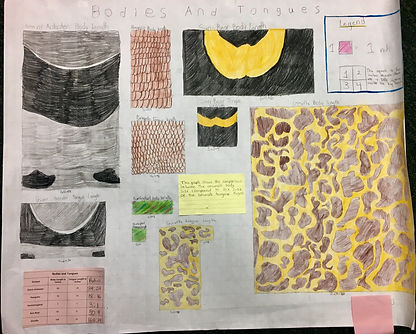top of page

BIG IDEA: Reasoning and Proportions

The work you see below builds on the work students did to interpret Animal Jump Graphs in a visualizing activity in math. Students thought about  animal jump graphs they studied previously as a jumping-off point for this activity. In this activity students were asked to think creatively about data sets, proportions and then display that data. They needed to make decisions about how to display data creatively while showing clarity and precision

Students used mathematical concepts such as measurement, intervals, area, data, proportions, ratios and ratio equivalents, factors and multiples.

Students then participated in a Gallery Walk  whereupon they provided feedback including:

Name something you observed (without judgement)

Name something you noticed that the mathematician did to make the graph easy to understand

Give the mathematician constructive feedback.

You can ask your child more about the math we are doing in 6A and use the photographs of their work below as a reference point.bottom of page